Next: singlet Up: Reference guide: Previous: polygon

### sine

NAME

sine

SYNOPSIS

          sine [-optional link arguments]
-x x0 -y y0 -d direction -p plength -l nump -a amplitude -h phase_shift


DESCRIPTION

sine creates a sinousoidal curve of immersed boundary points. sine uses two lines or arguments. The first line contains the optional link arguments. The second line contains the sine definitions.
• -x x0 - x coordinate of the sine start point (x0,y0)
• -y y0 - y coordinate of the sine start point (x0,y0)
• -d theta - direction in radians of the center line of the curve
• -p plength - period length of the wave
• -l nump - number of periods in the curve
• -a amplitude - amplitude of the sine wave
• -h phaseshift - phase shift of the wave at (x0,y0)
Using these definitions, sine defines the sinusoidal curve with the following formulas. Let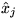be discrete points equally spaced in the interval [0,np] and let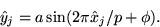Here a is the amplitude, p is the period length,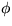is the phase shift and n is the number of periods. The immersed boundary points are found by a translation and rotation of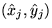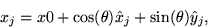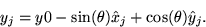sine allows for optional link arguments by interpreting the following options.

• -A name - indicates this entity is active and provides a name
• -a angle - resting angle at the IB points
• -b bend - bending stiffness at the IB points (default=40000)
• -d distance - initial distance between IB points (default=0.5)
• -e end tether - tether spring stiffness at the endpoints
• -N name - provides a name
• -n number - number of IB points around the circle
• -s stiff - inter-IB-point spring stiffness (default=40000)
• -t tether - tether spring stiffness (default=0)